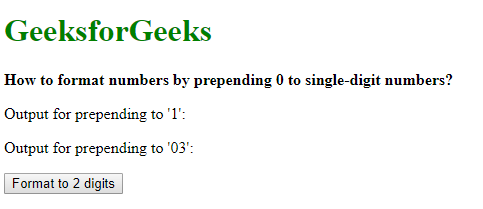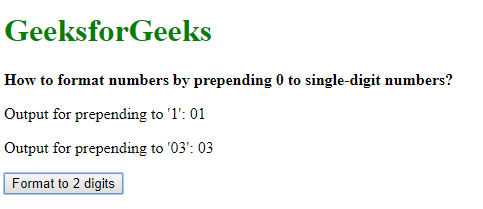# How to format numbers by prepending 0 to single-digit numbers?

A number can be formatted to prepend a 0 to single-digit numbers using 3 approaches:

The padStart() method is used to pad a string with another string to a certain length. The padding is started from the left of the string. It takes two parameters, the target length, and the string to be replaced with.

The number to be formatted is first converted to a string by passing it to the String constructor. The padStart() method is used on this string with the length parameter given as 2 and the string to be replaced with, given the character ‘0’. This will format any single digit number to 2 digits by prepending a ‘0’ and leave 2 digit numbers as is.

Syntax:

`prepended_number = String(number).padStart(2, '0')`

Example:

 ` ` `<``html``> ` ` `  `<``head``> ` `    ``<``title``> ` `      ``How to format numbers by  ` `      ``prepending 0 to single-digit numbers? ` `  `` ` ` ` ` `  `<``body``> ` `    ``<``h1` `style``=``"color: green"``> ` `      ``GeeksforGeeks ` `  `` ` `    ``<``b``>How to format numbers by  ` `      ``prepending 0 to single-digit numbers? ` `  `` ` `    ``<``p``>Output for prepending to '1': <``span` `class``=``"output"``> ` `      `` ` `  `` ` `    ``<``p``>Output for prepending to '03': <``span` `class``=``"output-2"``> ` `      `` ` `  `` ` ` `  `    ``<``button` `onclick``=``"padNumber()"``> ` `      ``Format to 2 digits ` `  `` ` `    ``<``script` `type``=``"text/javascript"``> ` `        ``function padNumber() { ` `            ``single_digit = 1; ` `            ``two_digits = 03; ` ` `  `            ``prepended_out =  ` `              ``String(single_digit).padStart(2, '0'); ` `            ``prepended_out2 =  ` `              ``String(two_digits).padStart(2, '0'); ` ` `  `            ``document.querySelector( ` `              ``'.output').textContent = prepended_out; ` `            ``document.querySelector( ` `              ``'.output-2').textContent = prepended_out2; ` `        ``} ` `    `` ` ` ` ` `  ` `

Output:

• Before clicking the button:• After clicking the button:Method 2: Checking if number is less than 9:
In this method, the number is first checked if it is less than 9. If true, the character ‘0’ is appended to the number otherwise, the number is returned without any change. This will format any single digit number to 2 digits by prepending a ‘0’ and leave 2 digit numbers as is.

Syntax:

```        function prependZero(number) {
if (number < 9)
return "0" + number;
else
return number;
}
```

Example:

 ` ` `<``html``> ` ` `  `<``head``> ` `    ``<``title``> ` `      ``How to format numbers by  ` `      ``prepending 0 to single-digit numbers? ` `  `` ` ` ` ` `  `<``body``> ` `    ``<``h1` `style``=``"color: green"``> ` `      ``GeeksforGeeks ` `  `` ` `    ``<``b``>How to format numbers by prepending ` `      ``0 to single-digit numbers? ` `    ``<``p``>Output for prepending to '1': <``span` `class``=``"output"``> ` `      `` ` `  `` ` `    ``<``p``>Output for prepending to '03': <``span` `class``=``"output-2"``> ` `      `` ` `  `` ` ` `  `    ``<``button` `onclick``=``"padNumber()"``> ` `      ``Format to 2 digits ` `  `` ` `    ``<``script` `type``=``"text/javascript"``> ` `        ``function prependZero(number) { ` `            ``if (number < ``9``) ` `                ``return "0" + number; ` `            ``else ` `                ``return number; ` `        ``} ` ` `  `        ``function padNumber() { ` `            ``single_digit` `= ``1``; ` `            ``two_digits` `= ``03``; ` ` `  `            ``prepended_out` `= ``prependZero``(single_digit); ` `            ``prepended_out2` `= ``prependZero``(two_digits); ` ` `  `            ``document.querySelector( ` `              ``'.output')``.textContent` `= ``prepended_out``; ` `            ``document.querySelector( ` `              ``'.output-2')``.textContent` `= ``prepended_out2``; ` `        ``} ` `    `` ` ` ` ` `  ` `

Output:

• Before clicking the button:• After clicking the button:Method 3: Using the slice() method:
The slice() method is used to extract parts of a string from the specified starting and ending indices. First, the number is prepended with a '0' character regardless of it being a single digit. This will make the single digit number into 2 digits but the 2-digit number would be converted to a 3-digit one with the extra '0'. The slice() method is used to extract the last 2 digits of the resulting number.

This will correctly get the last 2 digits of the 2-digit number discarding the extra '0' added to it. The single-digit number is now formatted with a '0'.

Syntax:prepended_number = ("0" + number).slice(-2)

Example:

 ` ` `<``html``> ` ` `  `<``head``> ` `    ``<``title``> ` `      ``How to format numbers by  ` `      ``prepending 0 to single-digit numbers? ` `  `` ` ` ` ` `  `<``body``> ` `    ``<``h1` `style``=``"color: green"``> ` `      ``GeeksforGeeks ` `  `` ` `    ``<``b``>How to format numbers by  ` `      ``prepending 0 to single-digit numbers? ` `  `` ` `    ``<``p``> ` `      ``Output for prepending to '1': <``span` `class``=``"output"``> ` `      `` ` `  `` ` `    ``<``p``> ` `      ``Output for prepending to '03': <``span` `class``=``"output-2"``> ` `      `` ` ` `  `    ``<``button` `onclick``=``"padNumber()"``> ` `      ``Format to 2 digits ` `  `` ` `    ``<``script` `type``=``"text/javascript"``> ` `        ``function padNumber() { ` `            ``single_digit = 1; ` `            ``two_digits = 03; ` ` `  `            ``prepended_out = ( ` `              ``"0" + single_digit).slice(-2); ` `            ``prepended_out2 = ( ` `              ``"0" + two_digits).slice(-2); ` ` `  `            ``document.querySelector( ` `              ``'.output').textContent = prepended_out; ` `            ``document.querySelector( ` `              ``'.output-2').textContent = prepended_out2; ` `        ``} ` `    `` ` ` ` ` `  ` `

Output:

• Before clicking the button:• After clicking the button:My Personal Notes arrow_drop_upCheck out this Author's contributed articles.

If you like GeeksforGeeks and would like to contribute, you can also write an article using contribute.geeksforgeeks.org or mail your article to contribute@geeksforgeeks.org. See your article appearing on the GeeksforGeeks main page and help other Geeks.

Please Improve this article if you find anything incorrect by clicking on the "Improve Article" button below.

Article Tags :

Be the First to upvote.

Please write to us at contribute@geeksforgeeks.org to report any issue with the above content.